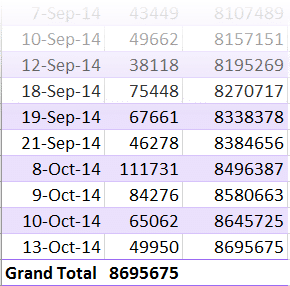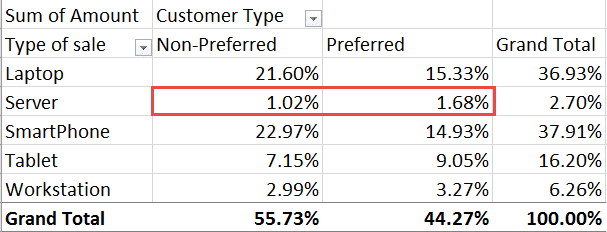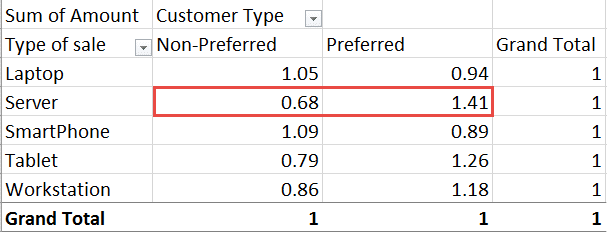# Pivot: Running Totals and Ranking

In this article we will see how to manage running totals and Ranking within Pivot Table calculations.## Recap

We are exploring the powerful built-in capabilities of Pivot Tables. Currently we are working on the Show Values As options.

## Running Totals

If your data is sorted by some date in rows, you may want running totals. Open the sample file – Drag Due Date into row area and amount in column area. Assume that date was the transaction date. Now we want to know the total value on each day. This is a running total.

Drag drop Amount column again and choose – Show Values As – Running Total In…
It will show the Due Date column automatically. Click Ok and then you have the running total shown.

If you see the last rows, notice that the Grand total in the first column is the running total in the second column. This option eliminates the need for adding formulas manually.Grand total is the 100% – it is the total of all values. Running total is showing the cumulative total. But if we see any intermediate running total, we cannot understand how much of the total accrual has happened at that point.

For example, if I see the highlighted value 1087879 for 5 may 2013, I know the cumulative total. But I still don’t know the grand total (without scrolling). So I still cannot understand how much of the amount was already accumulated as on that day.That is why in 2013, a new option was added % Running Total In…
Drag Drop amount in another column, right click and choose this option.
Click Ok for Due Date column and now you get this.1. Nice and easy. Don’t get confused.
2. First column is date.
3. Second column shows total transactions for that date.
4. Third column shows cumulative total as on that date.
5. Fourth column shows the cumulative total as a percentage of grand total.

Choose the option which suits your needs.

## Ranking

This is another common requirement – which we used to perform using the Rank() function. Now, (since 2013) it is a part of Show Values As.

Let us create a new pivot table. Drag Segment to rows, Drag Amount twice to data area. In the second amount column – Right Click – Show Values As – Rank Smallest to Largest
Choose Segment as the field.Lowest value gets rank ONE. You have an option to show the ranking in reverse order also. Usually we will only use the Ranking. This is a very simple way.

But now look at a dual field scenario. Here ranking is providing much more useful information. Here I chose “Type of Sale” as the field instead of “Segment”.Now it ranks Type of Sale within each Segment.

## Index option

This option is a big complex to understand. Let us look at this simple Pivot table. You can build this one quickly. Apply % of grand total from Show Values as to get this output.Now you can see how each value is contributing to overall business. Servers for both type of customers are contributing almost similar amount.

Now apply the Index function to the same data and see what happens. I have reduced the decimals to 2 digits to simplify data. You need not do that.Now notice the highlighted options. With % of total calculation, the difference between contribution of Preferred and Non-Preferred customers for Servers  was not very big.

But now look at the same INDEX values… The difference is much more pronounced.
Preferred is 1.41 and Non-Preferred is 0.68 – almost half.

Don’t worry about how index is calculated. The INDEX values give you better comparative results. That is why, it is preferred to the % of Grand Total. It gives you a more accurate representation of reality.

If you insist, here are the details of how Index is calculated.

## What Next?

Now we will shift focus to Calculations done using YOUR formulas within Pivot Table.

***